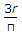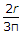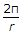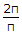# Civil Engineering - Applied Mechanics

### Exercise :: Applied Mechanics - Section 2

11.

The resultant of the forces acting on a body will be zero if the body

 A. rotates B. moves with variable velocity in a straight line C. moves along a curved path D. does not move at all.

Explanation:

No answer description available for this question. Let us discuss.

12.

A ball moving with a velocity of 5 m/sec impinges a fixed plane at an angle of 45° and its direction after impact is equally inclined to the line of impact. If the coefficient of restitution is 0.5, the velocity of the ball after impact will be

 A. 0.5 m/sec B. 1.5 m/sec C. 2.5 m/sec D. 3.5 m/sec E. 4.5 m/sec.

Explanation:

No answer description available for this question. Let us discuss.

13.

A pilot flies a small plane in a vertical loop of radius r. At the top of its trajectory he experiences weightlessness. If the acceleration due to gravity is g, the speed of the plane at the top of its trajectory would be

 A. zero B. infinite C. gr D. 2gr.

Explanation:

No answer description available for this question. Let us discuss.

14.

The distance of the c.g. of a semi-circular arc of radius r from its diameter along the radius of symmetry, is

 A.B.C.D.Explanation:

No answer description available for this question. Let us discuss.

15.

A Seconds pendulum executes

 A. 0.5 beat per second B. 1.0 beat per second C. 2.0 beats per second D. 2.5 beats per second E. 3 beats per second.